The Equilibrium Constant of Reactions

# The Equilibrium Constant of Reactions Notes | Study Additional Documents & Tests for Civil Engineering (CE) - Civil Engineering (CE)

## Document Description: The Equilibrium Constant of Reactions for Civil Engineering (CE) 2022 is part of Documents for Additional Documents & Tests for Civil Engineering (CE) preparation. The notes and questions for The Equilibrium Constant of Reactions have been prepared according to the Civil Engineering (CE) exam syllabus. Information about The Equilibrium Constant of Reactions covers topics like and The Equilibrium Constant of Reactions Example, for Civil Engineering (CE) 2022 Exam. Find important definitions, questions, notes, meanings, examples, exercises and tests below for The Equilibrium Constant of Reactions.

Introduction of The Equilibrium Constant of Reactions in English is available as part of our Additional Documents & Tests for Civil Engineering (CE) for Civil Engineering (CE) & The Equilibrium Constant of Reactions in Hindi for Additional Documents & Tests for Civil Engineering (CE) course. Download more important topics related with Documents, notes, lectures and mock test series for Civil Engineering (CE) Exam by signing up for free. Civil Engineering (CE): The Equilibrium Constant of Reactions Notes | Study Additional Documents & Tests for Civil Engineering (CE) - Civil Engineering (CE)
 1 Crore+ students have signed up on EduRev. Have you?

The Equilibrium Constant of Reactions

Since chemical composition of a reactive system undergoes change during a reaction, one may use the eqn. 6.41 for total differential of the Gibbs free energy change (for a single phase system):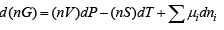..(6.41)

For simplicity considering a single reaction occurring in a closed system one can rewrite the last equation using eqn. 8.3: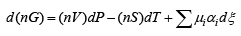..(8.40)

It follows that: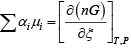..(8.41)

On further applying the general condition of thermodynamic equilibrium given by eqn. 6.36b it follows that: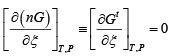...(8.42)

Hence by eqn. 8.41 and 8.42: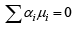...(8.43)

Since the reactive system is usually a mixture one may use the eqn. 6.123: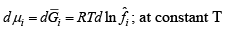...(6.123)

Integration of this equation at constant T from the standard state of species i to the reaction pressure: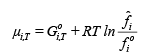...(8.44)

The ratio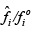is called the activity aˆi of species i in the reaction mixture, i.e.: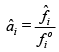...(8.45)

Thus, the preceding equation becomes: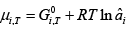..(8.46)

Using eqns. 8.46 and 8.44 in eqn. 8.43 to eliminate �i gives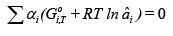...(8.47)

On further re-organization we have: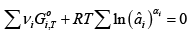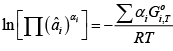...(8.48)

Where, ∏ signifies the product over all species i. Alternately: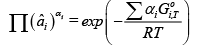...(8.49)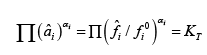...(8.50)

On comparing eqns. 8.49 and 8.50 it follows: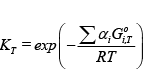...(8.51)

The parameter KT is defined as the equilibrium constant for the reaction at a given temperature. Since the standard Gibbs free energy of pure species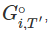depends only on temperature, the equilibrium constant KT is also a function of temperature alone. On the other hand, by eqn. 8.50 Kis a function of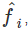which is in turn a function of composition, temperature and pressure. Thus, it follows that since temperature fixes the equilibrium constant, any variation in the pressure of the reaction must lead to a change of equilibrium composition subject to the constraint of Kremaining constant. Equation (8.51)  may also be written as: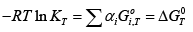...(8.52)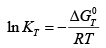...(8.53)

Taking a differential of eqn. 8.53: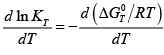...(8.53)

Now using eqn. 8.18: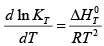...(8.54)

On further use of eqn. 8.13: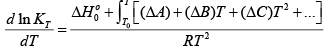Lastly, upon integration one obtains the following expression: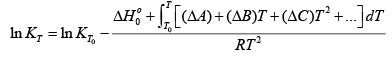...(8.55)

Where, KT0 is the reaction equilibrium constant at a temperature T0

If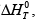is assumed independent of T (i.e.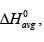, over a given range of temperature (T2 −T1) , a simpler relationship follows from eqn. 8.54: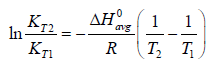...(8.55)

The above equation suggests that a plot of ln KT vs. 1/ T is expected to approximate a straight line. It also makes possible the estimation of the equilibrium constant at a temperature given its values at nother temperature. However,

eqn. 8.55 provides a more rigorous expression of the equilibrium constant as a function of temperature. Equation 8.54 gives an important clue to the variation of the equilibrium constant depending on the heat effect of the reaction. Thus, if the reaction is exothermic, i.e.,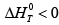, the equilibrium constant decreases with increasing temperature. On the other hand, if the reaction is endothermic, i.e.,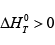, equilibrium constant increases with increasing temperature. As we shall see in the following section, the equilibrium conversion also follows the same pattern.

The document The Equilibrium Constant of Reactions Notes | Study Additional Documents & Tests for Civil Engineering (CE) - Civil Engineering (CE) is a part of the Civil Engineering (CE) Course Additional Documents & Tests for Civil Engineering (CE).
All you need of Civil Engineering (CE) at this link: Civil Engineering (CE)

## Additional Documents & Tests for Civil Engineering (CE)

62 docs|6 tests
 Use Code STAYHOME200 and get INR 200 additional OFF

## Additional Documents & Tests for Civil Engineering (CE)

62 docs|6 tests

Track your progress, build streaks, highlight & save important lessons and more!

,

,

,

,

,

,

,

,

,

,

,

,

,

,

,

,

,

,

,

,

,

;